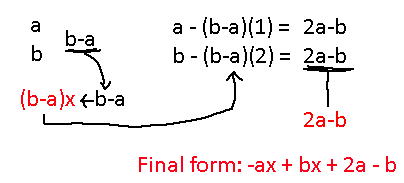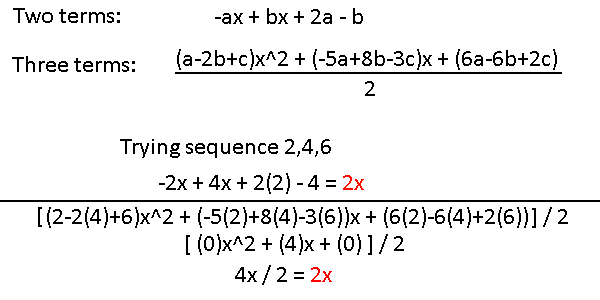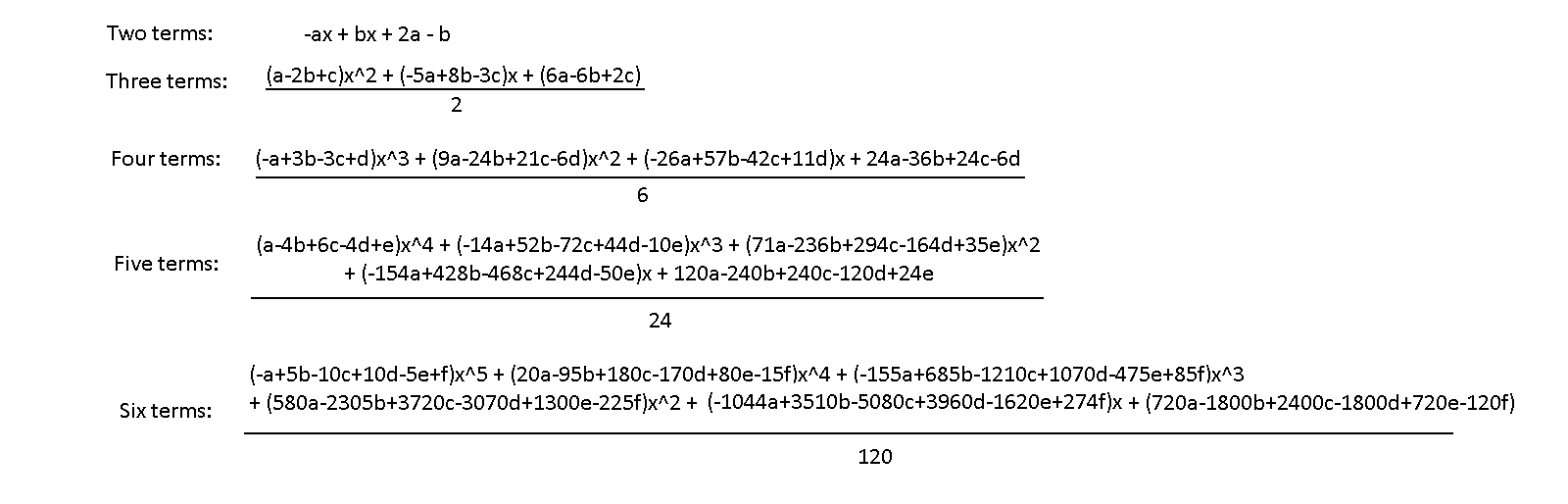# Polynomial Construction from Sequences II

This will build off the insights of the first post in this series. We now have a system for finding a polynomial that matches any sequence of numbers, but it would be nice if there was a faster way to apply this technique. If we substitute the sequence of numbers using variables we can run the whole process once, and end up with a short formula to push our sequence through!

The following is finding the polynomial for a two number sequence, except we make the numbers variables.That took a lot more work for 2 terms, but there it is! You can plug in any two number sequence and get back a formula which contains those numbers for x = 1 and x = 2. Looking at it more closely, we can see that -ax + bx determines the rate of change for the numbers, and that 2a - b gives the offset at which the sequence starts.

What does three terms look like? well…Even when taking shortcuts the process got a lot harder. In addition (or rather, subtraction), this process is highly susceptible to making mistakes.

So, we have these formulas. The three-term polynomial works similarly to the two-term polynomial, only it's able to take in an additional value so that it can find us polynomials of one higher order. In theory, we should be able to plug in a linear sequence like (2,4,6) to the three-term one and get back the same result as if we plugged in (2,4) to the first equation.There's something intriguing about watching these terms just cancelling out to 0. Think about this for a few moments: if you have a sequence where it was all just the same number (ex. 5,5,5,5,5), then plugging that in into any variation of these formulas will guarantee that all the higher order terms will cancel out, leaving you with the constant term at the end.

Look at the two-term formula: -ax + bx + 2a - b. Can you see how a constant sequence results in the higher order terms cancelling? Basically, a = b, so -ax + bx = 0 always and 2a - b = a, the value you started with. For the three-term formula, the values in the x^2 and x term will always cancel out: just add their coefficients together. A 'c' will always be left behind, which is also the starting value.

Furthermore, any sequence of numbers which increase or decrease the same amount every term will cause these formulas to cancel out all their values except for the x term and the constant term. If we look at the x^2 part in the three-term formula we can see why that's the case: a-2b+c. If you have three values which increase by the same amount, then it doesn't take much to show that the middle value is the average of the three, and that adding the first and third numbers gives you double the average.

I think it's really cool how so many combinations of patterns of numbers cause these terms to all cancel out. I'll show you some higher order formulas for larger sequences, but I will warn you now: it's about to get a lot more terrifying.Yikes. There are so many hidden patterns to attempt to extract from this that it's going to take yet another post to go through a fraction of it all. Perhaps there is an even better way to construct these formulas.